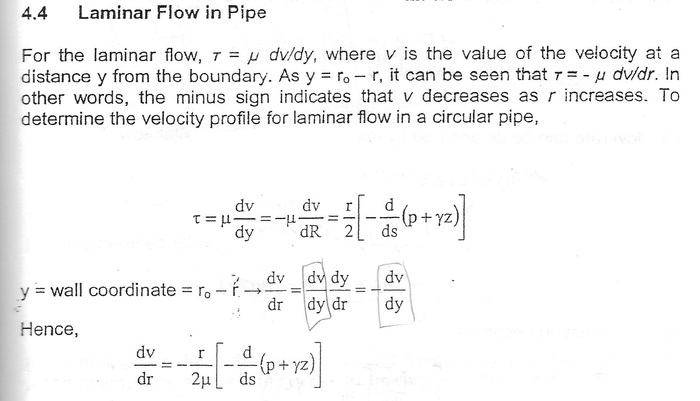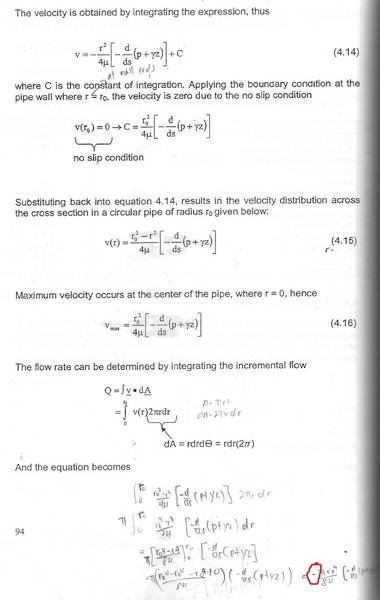# Equation of flow rate

## Homework Statement

the equation of flow aret given is [(pi)((r_o)^4 ) / 8μ ] [-d/ds (ρ +yz ) ] , but the ans that i got is - [(pi)((r_o)^4 ) / 8μ ] [-d/ds (ρ +yz ) ] , what's wrong with my working ?## The Attempt at a Solution

Try the simpler $$\int_0^{r_0} (r_0^2 -r^2)\, r dr$$to avoid things like the ##r_0^3## (?) in your first step
•Try the simpler $$\int_0^{r_0} (r_0^2 -r^2)\, r dr$$to avoid things like the ##r_0^3## (?) in your first step